## ¶ 1. 簡介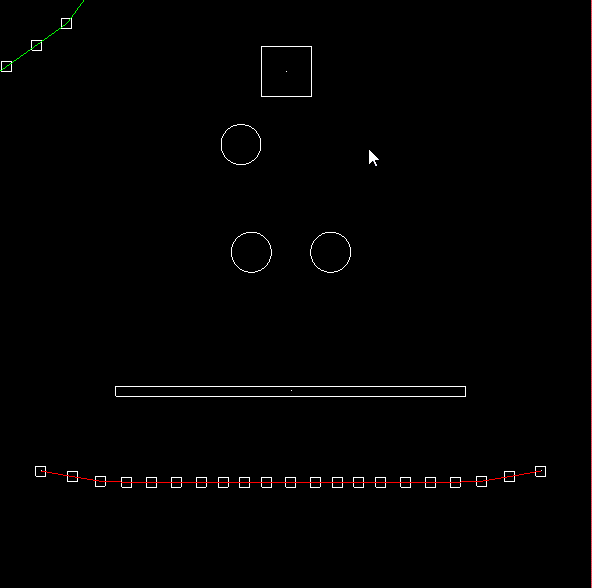## ¶ 2. 原理和實現

### ¶ 2.1 碰撞偵測

#### ¶ 2.1.1 AABB 與 AABB 碰撞

AABB 定義是沿著軸擺 (Axis-aligned)，也就是不考慮如果歪斜的形況。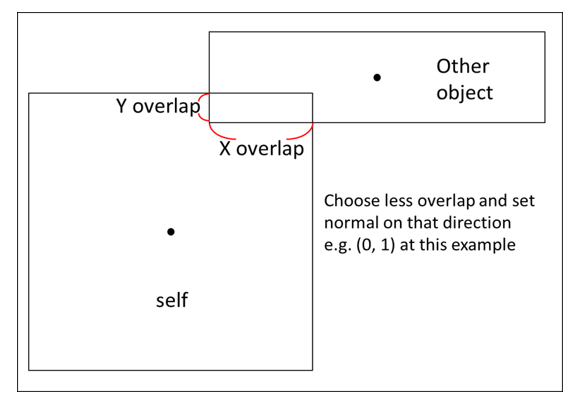$$penestration = min(\text {x overlap}, \text{y overlap})$$
$$\mathbf {Normal}_{BA} = \begin{cases} (1, 0) &\text{if min is x overlap} \\ (0, 1) &\text{if min is x overlap} \end{cases}$$

$$\begin{cases} A.{xmax} > B.{xmin} \\ B.{xmax} > A.{xmin}\\ A.{ymax} > B.{ymin} \\ B.{ymax} > A.{ymin} \end{cases}$$

typedef linalg::aliases::float2 float2;

float2 normal(0.0f, 0.0f);
float penetration = 0.0f;
bool is_hit = false;

if (A_x_max > B_x_min && B_x_max > A_x_min &&
B_y_max > B_y_min && B_y_max > B_y_min)
{
is_hit = true;
}

if (is_hit)
{
auto overlap_x = min(A_x_max - B_x_min, B_x_max - A_x_min);
auto overlap_y = min(B_y_max - B_y_min, B_y_max - B_y_min);

penetration = min(overlap_x, overlap_y);

if (penetration == overlap_x)
{
normal.x = B->pos.x - A->pos.x > 0 ? 1.0 : -1.0;
}
else
{
normal.y = B->pos.y - A->pos.y > 0 ? 1.0 : -1.0;
}
}


### ¶ 2.1.2 圓形與圓形碰撞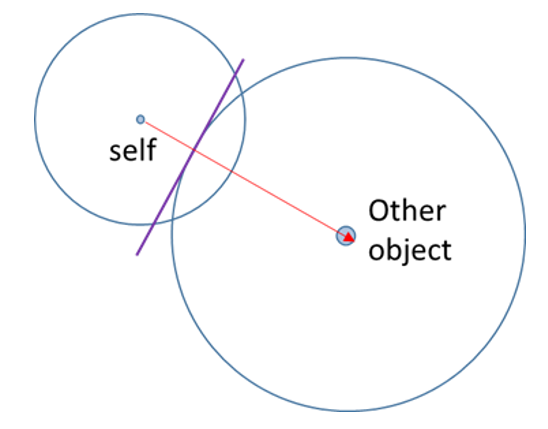$$penestration = R_A + R_B - Distance(C_A, C_B)$$
$$\mathbf {Normal}{BA} = \mathbf C{BA}/ Distance(C_A, C_B)$$

float2 normal(0.0f, 0.0f);
float penetration = 0.0f;
bool is_hit = false;

auto pos1 = A->pos;
auto pos2 = B->pos;

float dis = sqrt(
(pos2.x - pos1.x) * (pos2.x - pos1.x) +
(pos2.y - pos1.y) * (pos2.y - pos1.y));
penetration = r1 + r2 - dis;

if(penetration > 0) {
is_hit = true;
}

normal = float2((pos2.x - pos1.x) / dis, (pos2.y - pos1.y) / dis);


### ¶ 2.1.3 AABB 與圓形的碰撞

AABB 與圓形碰撞就比較複雜，在求穿透量和單位正向向量時情況分兩種，一種是碰撞後圓心在 AABB 之外，另一種是圓心在 AABB 之內，計算方法會有點差別。

#### ¶ 2.1.3.1 圓心在 AABB 之外

，而正向量則為綠色向量減掉藍色向量。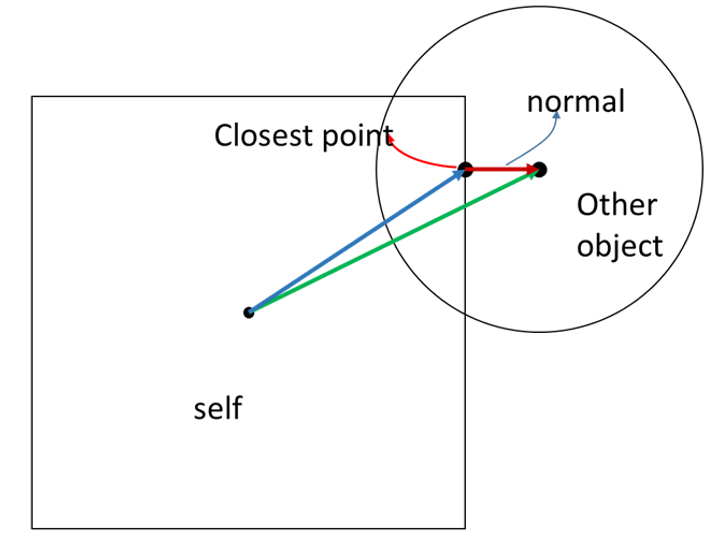$$\mathbf N_{BA} = \mathbf V_{B_{center}A_{center}} - \mathbf V_{PA_{center}}$$
$$penestration = B_{radius} - Distance(B_{center}, P)$$

float2 normal(0.0f, 0.0f);
float penetration = 0.0f;
bool is_hit = false;

auto ab_pos = A->pos; // AABB center
auto ab_ext = A->extent / 2;
auto x_max = ab_pos.x + ab_ext.x;
auto x_min = ab_pos.x - ab_ext.x;
auto y_max = ab_pos.y + ab_ext.y;
auto y_min = ab_pos.y - ab_ext.y;

auto c_pos = B->pos; // circle center

float2 closest_p(0, 0);

// outside
if (c_pos.x > x_max && c_pos.x < x_min &&
c_pos.y > y_max && c_pos.y < y_min)
{
closest_p.x = clamp(c_pos.x, x_min, x_max);
closest_p.y = clamp(c_pos.y, y_min, y_max);

float dis = sqrt(
(c_pos.x - closest_p.x) * (c_pos.x - closest_p.x) +
(closest_p.y - c_pos.y) * (closest_p.y - c_pos.y));

if (penetration > 0)
{
is_hit = true;
normal.x = (c_pos.x - closest_p.x) / dis;
normal.y = (c_pos.y - closest_p.y) / dis;
}
}


#### ¶ 2.1.3.2 圓心在 AABB 之內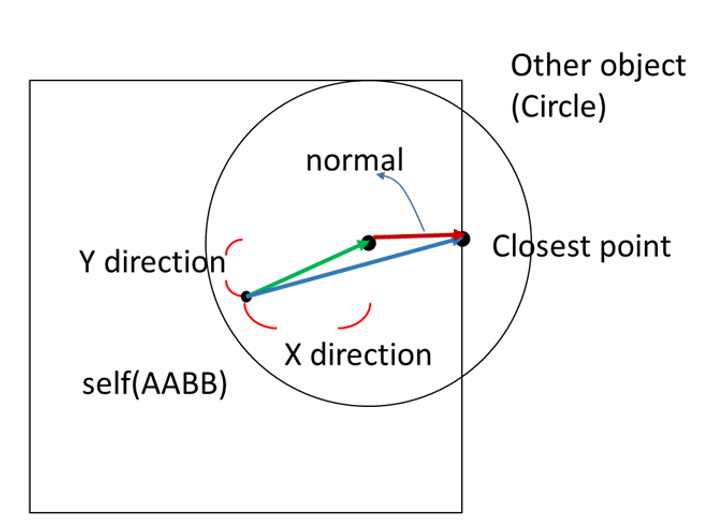$$\mathbf N_{BA} = \mathbf V_{PA_{center}} - \mathbf V_{B_{center}A_{center}}$$
$$penestration = B_{radius} + Distance(B_{center}, P)$$

// inside
else
{
auto x_right = x_max - c_pos.x;
auto x_left = c_pos.x - x_min;
auto y_up = y_max - c_pos.y;
auto y_down = c_pos.y - y_min;

auto smallest = MIN(x_right, x_left, y_up, y_down)

if (smallest == x_right)
{
closest_p.x = x_max;
closest_p.y = c_pos.y;
}
else if (smallest == x_left)
{
closest_p.x = x_min;
closest_p.y = c_pos.y;
}
else if (smallest == y_down)
{
closest_p.x = c_pos.x;
closest_p.y = y_min;
}
else
{

closest_p.x = c_pos.x;
closest_p.y = y_max;
}

float dis = sqrt(
(c_pos.x - closest_p.x) * (c_pos.x - closest_p.x) +
(closest_p.y - c_pos.y) * (closest_p.y - c_pos.y));

is_hit = true;
normal.x = (closest_p.x - c_pos.x) / dis;
normal.y = (closest_p.y - c_pos.y) / dis;
}


#### ¶ 2.1.4 位置修正

void correct_position(A, B)
{
const float percent = 0.2 // 60 fps 下通常 20% 到 80%
const float slop = 0.01 // 超過一定量才做修正，避免一直跳動，通常 0.01 to 0.1
float2 correction = max(penetration - slop, 0.0f) / (A.inv_mass + B.inv_mass)) * percent * normal
A.position -= A.inv_mass * correction
B.position += B.inv_mass * correction
}


### ¶ 2.2 動量衝量與摩擦力

#### ¶ 2.2.1 動量衝量處理

$$\mathbf J_r = \frac{-(1 + e)((\mathbf V^B - \mathbf V^A) \cdot\mathbf n)}{\frac{1}{mass^A} + \frac{1}{mass^B}}$$

void Resolve(body0, body1) {
if (isHit)
{
auto rel = body1->velocity - body0->velocity;
auto vel_n = dot(rel, normal);

// seperate
if (vel_n > 0)
{
return;
}

// restitution
float e = min(body0->restitution, body1->restitution);

// impluse
float jr = -(1 + e) * vel_n;
jr /= body0->inv_mass + body1->inv_mass;
auto impulse = jr * normal;

// Apply impulse
body0->SetVelocity(body0->velocity - body0->inv_mass * impulse);
body1->SetVelocity(body1->velocity + body1->inv_mass * impulse);

/*
處理摩擦力的部分
*/
}
}


#### ¶ 2.2.2 摩擦力處理

$$\mathbf V^R = \mathbf V^{B}-\mathbf V^{A} \ \mathbf V^R_{tangent} =\mathbf V^R - (\mathbf V^R \cdot \mathbf n) *\mathbf n$$

$$\mathbf Jt = \mathbf V^R \cdot {\mathbf V^R_{tangent}\over \Vert\mathbf V^R_{tangent}\Vert}$$

// 摩擦衝量

rel = body1->velocity - body0->velocity;
auto rel_normal = dot(rel, normal) * normal;
float2 rel_tan = rel - rel_normal;
float len = sqrt(rel_tan.x * rel_tan.x + rel_tan.y * rel_tan.y);

float2 tan(0, 0);
// 避免除以 0
if (len > 0.00001) {
tan /= len;
}

auto jt = -dot(rel, tan);
jt /= body0->inv_mass + body1->inv_mass;

float2 friction_impulse(0.0f, 0.0f);
float mu = sqrt(
pow(body0->StaticFriction, 2) + pow(body1->StaticFriction, 2));

// 靜摩擦
if (abs(jt) < jr * mu)
{
friction_impulse = jt * tan;
}
// 動摩擦
else
{
mu = sqrt(
pow(body0->DynamicFriction, 2) + pow(body1->DynamicFriction, 2));
friction_impulse = -jr * tan * mu;
}

// 計算摩擦衝量造成的速度差
body0->SetVelocity(body0->velocity - body0->inv_mass * friction_impulse);
body1->SetVelocity(body1->velocity + body1->inv_mass * friction_impulse);


### ¶ 2.3 連結限制 (Joint Constraint)

#### ¶ 2.3.1 彈簧力 (Spring Force)

\begin{align} \mathbf F_a &= -K_s(\vert \mathbf x_a - \mathbf x_b \vert - length)\mathbf L&, K_s > 0 \\ &= -K_s(\vert \mathbf x_a - \mathbf x_b \vert - length){\mathbf x_a - \mathbf x_b \over{\vert \mathbf x_a - \mathbf x_b \vert}}&, K_s > 0 \end{align}

auto pos_vec = body0->pos - body1->pos;
auto dis = sqrt(pow(pos_vec.x, 2) + pow(pos_vec.y, 2));

float2 f0(0.0f, 0.0f);
float2 f1(0.0f, 0.0f);

// 避免除以 0
if (dis > 0.00001)
{
f0 = -ks * (dis - length) * pos_vec / dis;
f1 = ks * (dis - length) * pos_vec / dis;
}

body0->force += f0
boddy1->force += f1


#### ¶ 2.3.2 阻尼力 (Damper Force)

\begin{align} \mathbf F_a &= -K_s((\mathbf v_a - \mathbf v_b ) \cdot \mathbf L)\mathbf L&, K_d > 0 \\ &= -K_s{(\mathbf v_a - \mathbf v_b)\cdot(\mathbf x_a - \mathbf x_b) \over{\vert \mathbf x_a - \mathbf x_b \vert}}{\mathbf x_a - \mathbf x_b \over{\vert \mathbf x_a - \mathbf x_b \vert}}&, K_s > 0 \end{align}

auto pos_vec = body0->pos - body1->pos;
auto dis = sqrt(pow(pos_vec.x, 2) + pow(pos_vec.y, 2));
auto v_vec = body0->velocity - body1->velocity;

float2 f0(0.0f, 0.0f);
float2 f1(0.0f, 0.0f);
if (dis > 0.00001)
{
f0 = -kd * (dis - lenght) * pos_vec / dis;
f1 = kd * (dis - length) * pos_vec / dis;
}

body0->force += f0
boddy1->force += f1


#### ¶ 2.3.3 固定長度連結 (Distance Joint)

auto pos_vec = body0->pos - body1->pos;
auto dis = sqrt(pow(pos_vec.x, 2) + pow(pos_vec.y, 2));
auto rel_dis = dis - length;

// 如果超過長度限制
if (rel_dis > 0)
{
// 位置修正
float2 unit_axis = pos_vec / dis;
body1->pos = body0->pos + unit_axis * length;

auto rel_vel = dot((body1->velocity - body0->velocity, unit_axis);
auto remove = rel_vel + rel_dis / deltaTime;
auto impulse = remove / (body0->inv_mass + body1->inv_mass);
auto impulse_vec = unit_axis * impulse;
auto force = impulse_vec / deltaTime;

body0->force -= force;
boddy1->force += force;
}


### ¶ 2.4 數值方法

#### ¶ 2.4.1 尤拉法

void timeStep(body) {
body->velocity += body->force * delta_time / body->mass; // Ft = mv
body->pos += delta_time * vel;
}


#### ¶ 2.4.2 Runge-Kutta 方法

Runge-Kutta 法的數學推導請參閱維基百科 Runge–Kutta methods

void timeStep(body) {
auto f = [=](StateStep &step, float2 vel, float t) {
vel += gravity * t;
step.velocity = vel;
};

StateStep F0, F1, F2, F3, F4;
F0.velocity = float2(0,0);
f(F1, F0.velocity, 0);
f(F2, F1.velocity / 2, deltaTime / 2);
f(F3, F2.velocity / 2, deltaTime / 2);
f(F4, F3.velocity, deltaTime);

auto v0 = body->vel + body->force * deltaTime / body->mass;
body->vel =  v0 + (F1.velocity + 2 * F2.velocity + 2 * F3.velocity + F4.velocity) / 6;
body->pos += body->vel * deltaTime;
}


## ¶ 3. 結果與討論

### ¶ 3.3 尤拉法和 Runge-Kutta 比較

Runge-Kutta 法可以是很高維的方法，稱之為 n-th RK method，但為求取計算準度和計算速度之間平衡，通常取四階，又稱為 RK 4th。RK 4th 取四種計算出來的結果，做加權平均，在處理四階以內的微分方程都會有很不錯的表現，同時因為他對步距大小 (Delta) 的敏感度比較低，所以模擬時候可以把步距設大一點，在算得比較快的同時，也能得到比較準確的數值解。